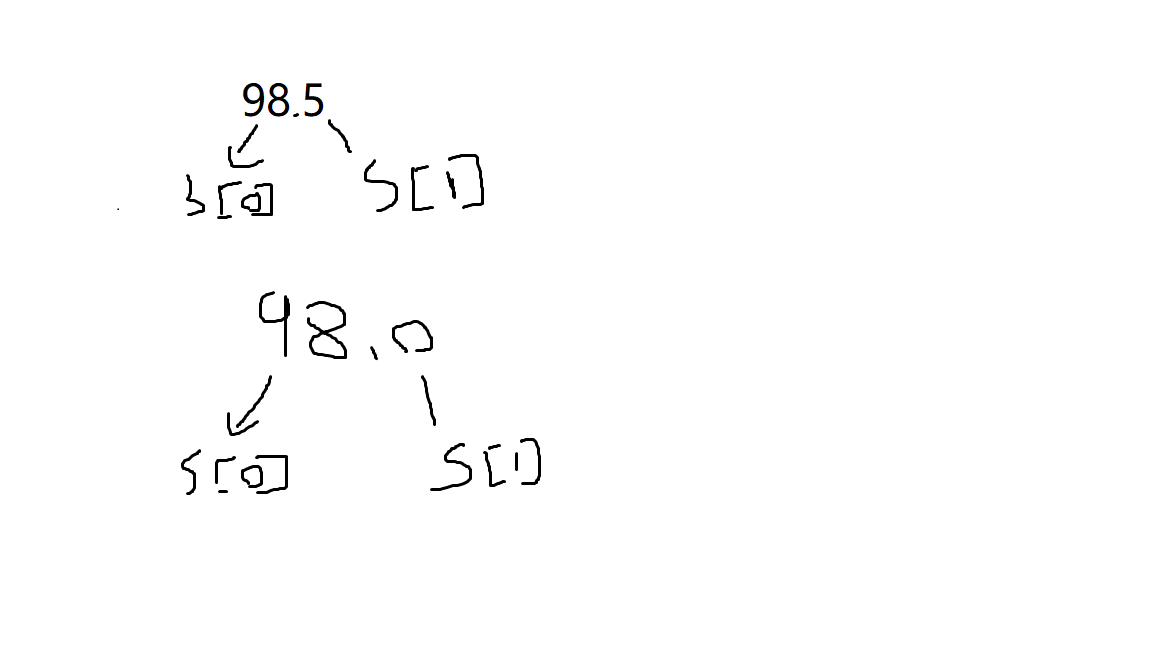• python判断整数还是小数
千次阅读
2020-12-03 15:58:19

# python判断整数、小数

g = input(“请输入判断的数：”)
a = str(g).split(’.’)
b = float(g)
c = ‘’.join(a)
if b == d:
print(“整数”)
else:
print(“小数”)python
更多相关内容
• 做题时快速判断一个数是否是小数
a=98.5
s=str(a).split('.')
if float(s)==0:
print("整数")
else:
print("小数")

数转化为字符串并通过split在小数点处分割形成一个列表转化为浮点型后我们只需要判断s是否为0就可以看出a是否为小数了。

对于直接的一个数进行判断这确实繁琐，但是对于一个不确定的数进行判断能较为快速编码。

展开全文python
• 输入例子：1.2，-1.2，.2,-2. def is_float(i): if i.count('.') == 1:#先判断... right = new_i[-1]#将小数分为小数点右边 left = new_i#小数点左边 if right.isdigit():#如果小数点右边是数字判断小数点左边

1.今天遇到一个问题如果输入的是字符串还是整数或者是小数如何将他们区分

首先isdigit()只能用来判断字符串输入的是否是整数，无法判断是否是小数

所以，先判断该字符串是否是整数，如果是返回3，

不是的话说明是字母或者是小数，然后判断是否是小数，如果是小数的话返回1，

是字母的或其他的话返回2

def is_float(i):
if i.isdigit():#只能用来判断整数的字符串
return  3
else:
if i.count('.') == 1:  # 先判断里面有没有小数点
new_i = i.split('.')  # 去掉小数点
right = new_i[-1]  # 将小数分为小数点右边
left = new_i  # 小数点左边
if right.isdigit():  # 如果小数点右边是数字判断小数点左边
if left.isdigit():  # 如果小数点左边没有-直接返回
return 1
elif left.count('-') == 1 and left.startswith('-'):  # 如果小数点左边有-
new_left = left.split('-')[-1]  # 判断去掉后的还是不是数字
if new_left.isdigit():  # 是数字则返回True
return 1
else:
return 2  # 返回2说明是字母

输入例子：1.2，-1.2，.2,-2.

def is_float(i):
if i.count('.') == 1:#先判断里面有没有小数点
new_i = i.split('.')#去掉小数点
right = new_i[-1]#将小数分为小数点右边
left = new_i#小数点左边
if right.isdigit():#如果小数点右边是数字判断小数点左边
if left.isdigit():#如果小数点左边没有-直接返回
return True
elif left.count('-')== 1 and left.startswith('-'):#如果小数点左边有-
new_left = left.split('-')[-1]#判断去掉后的还是不是数字
if new_left.isdigit():#是数字则返回True
return True
else:
return False

更简单的判断方法：

while  True:
num = input("请输入一个数字:")
try:
n1=eval(num)
except:
print("输入的不是数字程序结束")
break

if isinstance(n1,float):
print('输入的是小数请重新输入:')
continue
else:
print("输入的是整数没问题")

展开全文python
• python 判断是否为正小数和正整数的实例 实现代码： def check_float(string): #支付时，输入的金额可能是小数，也可能是整数 s = str(string) if s.count('.') == 1: # 判断小数点个数 sl = s.split('.') # ...
• ## Python

千次阅读 2021-01-29 13:08:29
Python 判断是否为整数Python 有默认的整数判断函数str.isdigit()，但其无法判断整数，因此需要先把负整数转化为无符号的整数代码示例：@classmethoddef is_number(cls, numStr):''' 字符串是否是整数'''flag = ...

Python 判断是否为整数

Python 有默认的整数判断函数str.isdigit()，但其无法判断负整数，因此需要先把负整数转化为无符号的整数

代码示例：

@classmethod

def is_number(cls, numStr):

''' 字符串是否是整数

'''

flag = False

numStr = str(numStr).strip().lstrip('-').lstrip('+') # 去除正数(+)、负数(-)符号

if str(numStr).isdigit():

flag = True

return flag

补充知识：

str为字符串

str.isalnum() 所有字符都是数字或者字母

str.isalpha() 所有字符都是字母

str.isdigit() 所有字符都是数字(不能判断正数(+)、负数(-)符号)

str.islower() 所有字符都是小写

str.isupper() 所有字符都是大写

str.istitle() 所有单词都是首字母大写

str.isspace() 所有字符都是空白字符、\t、\n、\r

Python 判断是否为小数

Python 判断小数至少有三种方法，分别如下

方法1：正则匹配 re

@classmethod

def is_float(cls, numStr):

''' 字符串是否是浮点数(整数不算小数)

'''

flag = False

numStr = str(numStr).strip().lstrip('-').lstrip('+') # 去除正数(+)、负数(-)符号

try:

reg = re.compile(r'^[-+]?[0-9]+\.[0-9]+$') res = reg.match(str(numStr)) if res: flag = True except Exception as ex: print("is_float() - error: " + str(ex)) return flag 正则表达式说明： '^[-+]?[0-9]+\.[0-9]+$'

^表示以这个字符开头，也就是以[-+]开头，[-+]表示字符-或者+

?表示0个或1个，也就是说符号+和1是可选的，也可以没有。

[0-9]表示0到9的一个数字，+表示1个或多个，也就是整数部分。

\.表示的是小数点，\是转义字符因为.是特殊符号(匹配任意单个除\r\n之外的字符)，所以需要转义。

小数点后的也需要是数字，

$表示字符串以此结尾。 整个匹配中不可以出现非数字或其他符号。 方法2：浮点转化，异常判断 @classmethod def is_float2(cls, numStr): ''' 字符串是否是浮点数(整数算小数) ''' flag = False numStr = str(numStr).strip().lstrip('-').lstrip('+') # 去除正数(+)、负数(-)符号 try: numFloat = float(numStr) flag = True except Exception as ex: print("is_float() - error: " + str(ex)) return flag 方法3：逐个字符判断是否为数字或小数点 @classmethod def is_float3(cls, numStr): ''' 字符串是否是浮点数(整数算小数) ''' flag = True numStr = str(numStr).strip().lstrip('-').lstrip('+') # 去除正数(+)、负数(-)符号 floatNum = string.digits + "." for i in range(len(numStr)): if numStr[i] in floatNum: continue else: flag = False return flag 测试代码 if __name__ == '__main__': numStr = 23 print(numStr, " : ", YGDTime.is_number(numStr), " : ", YGDTime.is_float(numStr)) numStr = '23' print(numStr, " : ", YGDTime.is_number(numStr), " : ", YGDTime.is_float(numStr)) numStr = -23 print(numStr, " : ", YGDTime.is_number(numStr), " : ", YGDTime.is_float(numStr)) numStr = -23.123 print(numStr, " : ", YGDTime.is_number(numStr), " : ", YGDTime.is_float(numStr)) numStr = '23aw' print(numStr, " : ", YGDTime.is_number(numStr), " : ", YGDTime.is_float(numStr)) 运行结果： (23, ' : ', True, ' : ', False) ('23', ' : ', True, ' : ', False) (-23, ' : ', True, ' : ', False) (-23.123, ' : ', False, ' : ', True) ('23aw', ' : ', False, ' : ', False) 参考推荐： 展开全文• 输入字符串，输出对应的整数小数、布尔值或字符串。python • 一、判断一个数是否为小数1、有且仅有一个小数点2、小数点的左边可能为正数或负数3、小数点的右边为正数二、实现代码def is_float(str):if str.count('.') == 1: #小数有且仅有一个小数点left = str.split('.') #... • python 匹配整数或者小数（包括正数和负数）（简单易懂，代码可以直接运行） *这个实验算是五个正则表达式里面最难的的哪一个了，？是正则表达式里面贪婪与非贪婪的概念，有？则-？可有可无，刚好可以用于判断正数和...python 正则表达式 • import re def judge_number(str): regInt = '^0$|^[1-9]\d*\$' # 不接受09这样的为整数 ... regIntOrFloat = regInt + '|' + regFloat # 整数小数 patternIntOrFloat = re.compile(regIntOrFloat) i...python
• ## python判断整数

千次阅读 2020-11-20 20:03:47
python输出整数的方法：先使用str()函数将数字转换成字符串赋值给变量i，再用“if i.count(‘.’) == 0”语句判断字符串中是否没有小数点，如果是则输出这个字符串，这样输出的数字就都是整数了示例代码如下：x = ...
• python如何判断元素是否为小数发布时间：2020-09-24 15:48:31来源：亿速云阅读：79作者：Leah这篇文章将为大家详细讲解有关python如何判断元素是否为小数，文章内容质量较高，因此小编分享给大家做个参考，希望大家...
• ## 判断整数python

千次阅读 2020-11-23 11:01:35
均已开源，支持 python、java、php、go、nodejs、.net、c++。 以云服务器查看实例列表(describeinstances)请求为例，当用户调用这一接口时，其请求参数可能如下:参数名称中文参数值 action方法名describeinstances ...python判断整数
• def is_number(s): if s.count(".") == 1 and s[-1] != "%": # 小数判断 if s == "-": s = s[1:] if s == ".": return False s = s.replace(".", "") for i in s: if i not in "0123456789":python
• 方法1 判断是否为整数（即使不为整型） # -*- coding: utf-8 -*- """ @File : test.py @Time : 2020/6/25 11:27 @Author : Dontla @Email : sxana@qq.com @Software: PyCharm """ a = 1.004 b = 0.00 c = 0.543 d = ...
• Python判断一个数是否为小数一、判断一个数是否为小数1、有且仅有一个小数点2、小数点的左边可能为正数或负数3、小数点的右边为正数二、实现代码def is_float(str):if str.count('.') == 1: #小数有且仅有一个小数点...
• Python|如何判断数字是否为小数 函数介绍 自定义一个用于判断数字是否为小数的函数： 若为小数则输出该数字，若为整数则输出整数形式（int） 创作背景 在进行除法计算时，结果以浮点数表示（如下图），不方便判断...python
• 但是字符串没有内置判断小数的方法，所以我们动手写一个判断字符串是否是小数，可以判断正负小数，代码如下：'''1、判断小数点个数是不是1 count2、分割，判断小数点右边是不是整数3、判断小数点左边是整数is_...
• 今天写vue统计列表显示进度格式为：32%或34.34%，前端实现保留两位小数整数不处理）直接用了内置方法 parseFloat(((pass_num + fail_num + na_num)/case_num *100).toFixed(2)) 有个列表写的时候不支持后端拿出来...python
• 在能将浮点数准确转化为浮点数后，基于字符串处理是非常容易获得后面的小数位置的，这里不做过多介绍。包进行处理即可，今天这个需求有点懵，搜索相关资料，在不改变小数位的情况下，可以有两种解决方案。这样就不能...python 字符串 float
• 数字用isdigit()方法 小数用eval()和isinstance()结合 print('100'.isdigit()) print(isinstance(eval('1.1',float))python
• 猛一看整数和整型没有区别，所以网上有一堆使用使用isinstance来判断一个数是否是整数，但是我们在console中输入如下实例时，会发现： >>>isinstance(2,int) True >>>isinstance(2.0,int) False ...判断整数 python判断整数 python判断整型 isinstance
• Python解释器的源码里看到一段有趣的代码，它实现了Python两个整数相加时如果溢出则用更大的数据类型保存整数。具体代码的如下。// Python解释器是C语言实现的static PyObject *int_add(PyIntObject *v, ...
• a=input() x,y=a.split (’.’) print(‘整数{}小数{}’.format(x,int(y)//1))
• 首先是解决我问题的方法,使用isinstance(i,float/int)判断： i=9，j=1.3 &gt;&gt;isinstance(i,int) &gt;&gt;True &gt;&gt;isinstance(i,float) &gt;&gt;False &gt;&gt;...python
• a=81**0.5 s=str(a).split('.') if float(s)==0: print('整数') else :print('小数') 转载于:https://www.cnblogs.com/mghhzAnne/p/11151766.html
• 一、整数类型(int)整数就是没有小数部分的数字，Python中的整数包括正整数、0 和负整数。有些强类型的编程语言会提供多种整数类型，每种类型的长度都不同，能容纳的整数的大小也不同，开发者要根据实际数字的大小......